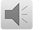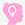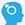# 多重检验中为何计算Bonferroni校正P值？

•时间：2021-01-02   阅: 9073
标签: 统计方法   多重检验多重检验中为何计算Bonferroni校正P值?SCI投稿返修，reviewer让其计算Bonferroni校正P值。而我们知道，Bonferroni校正是统计学上减少I类错误的方法。

常规我们关心的Bonferoni校正，是指对检验水准alpha进行校正，如三组比较，两两比较需要比较三次，则校正检验水准alpha=0.05/3=0.0167，只有P<0.0167，我们才认为两组间有差异，这就是Bonferroni校正

可是Reviewer让计算Bonferroni校正P值是咋回事呢?我们先看看英文原文是怎么解读的吧。

Problem:How does SPSS calculate the Bonferroni-corrected p-valuesfor pairwise comparisons?

Resolving the problem.

SPSS offers Bonferroni-adjusted significance tests for pairwise comparisons. This adjustment is available as an option for post hoc tests and for the estimated marginal means feature.

Statistical textbooks often present Bonferroni adjustment (or correction) inthe following terms. First, divide the desired alpha-level by the number of comparisons. Second, use the number so calculated as the p-value for determining significance. So, for example, with alpha set at .05, and three comparisons, the LSD p-value required for significance would be .05/3 =.0167.

SPSS and some other major packages employ a mathematically equivalent adjustment. Here's how it works. Take the observed (uncorrected) p-value and multiply it by the number of comparisons made. What does this mean in the context of the previous example, in which alpha was set at .05 and there were three pairwise comparisons? It's very simple. Suppose the LSD p-value for a pair wise comparison is .016. This is an unadjusted p-value. To obtain the corrected p-value, we simply multiply the uncorrected p-value of .016 by 3, which equals.048. Since this value is less than .05, we would conclude that the difference was significant.

Finally, it's important to understand what happens when the product of the LSD p-value and the number of comparisons exceeds 1. In such cases, the Bonferroni-corrected p-value reported by SPSS will be 1.000. The reason for this is that probabilities cannot exceed 1. With respect to the previous example, this means that if an LSD p-value for one of the contrasts were .500,the Bonferroni-adjusted p-value reported would be 1.000 and not 1.500, which isthe product of .5 multiplied by 3

以上大家看明白了吗，其实Bonferroni校正挺简单，就是用我们分析的两两比较的原始P值去乘以比较的次数，得到的P值如果<0.05，就说明差异有统计写意义。

比如，3组资料比较有统计学意义，现在要两两比较3次。某一次比较的原始P值=0.016。则Bonferroni校正P=0.016×3=0.048<0.05，因此该两组间差异有统计学意义。

如果上述的另两组原始比较P=0.025，虽然是小于0.05，但0.025×3=0.075>0.05，因此，该两组的差异尚不能认为有统计学意义。

Multiple testing corrections adjust p-values derived from multiple statistical tests to correct for occurrence of false positives. In microarray data analysis, false positives are genes that are found to be statistically different between conditions, but are not in reality.

方法：A. Bonferroni correction

The p-value of each gene is multiplied by the number of genes in the gene list. If the corrected p-value is still below the error rate, the gene will be significant:

Corrected P-value= p-value * n (number of genes in test) <0.05

As a consequence, if testing 1000 genes at a time, the highest accepted individual pvalue is 0.00005, making the correction very stringent. With a Family-wise error rate of 0.05 (i.e., the probability of at least one error in the family), the expected number of false positives will be 0.05.

B. Bonferroni Step-down (Holm) correction

This correction is very similar to the Bonferroni, but a little less stringent:

1) The p-value of each gene is ranked from the smallest to the largest.

2) The first p-value is multiplied by the number of genes present in the gene list:

if the end value is less than 0.05, the gene is significant:

Corrected P-value= p-value * n < 0.05

3) The second p-value is multiplied by the number of genes less 1:

Corrected P-value= p-value * n-1 < 0.05

4) The third p-value is multiplied by the number of genes less 2:

Corrected P-value= p-value * n-2 < 0.05

It follows that sequence until no gene is found to be significant.

Example:

Let n=1000, error rate=0.05

Gene

name

p-value before

correction

Rank Correction Is gene significant

after correction?

A 0.00002 1 0.00002 * 1000=0.02 0.02<0.05 => Yes

B 0.00004 2 0.00004*999=0.039 0.039<0.05 => Yes

C 0.00009 3 0.00009*998=0.0898 0.0898>0.05 => No

Because it is a little less corrective as the p-value increases, this correction is less

conservative. However the Family-wise error rate is very similar to the Bonferroni

correction (see table in section IV).

C. Westfall and Young Permutation

Both Bonferroni and Holm methods are called single-step procedures, where each p value is corrected independently. The Westfall and Young permutation method takes advantage of the dependence structure between genes, by permuting all the genes at the same time.

The Westfall and Young permutation follows a step-down procedure similar to the Holm method, combined with a bootstrapping method to compute the p-value distribution:

1) P-values are calculated for each gene based on the original data set and ranked.

2) The permutation method creates a pseudo-data set by dividing the data into artificial treatment and control groups.

3) P-values for all genes are computed on the pseudo-data set.

4) The successive minima of the new p-values are retained and compared to the original ones.

5) This process is repeated a large number of times, and the proportion of resampled data sets where the minimum pseudo-p-value is less than the original p-value is the adjusted p-value.

Because of the permutations, the method is very slow. The Westfall and Young permutation method has a similar Family-wise error rate as the Bonferroni and Holm corrections.

D. Benjamini and Hochberg False Discovery Rate

This correction is the least stringent of all 4 options, and therefore tolerates more false positives. There will be also less false negative genes. Here is how it works:

1) The p-values of each gene are ranked from the smallest to the largest.

2) The largest p-value remains as it is.

3) The second largest p-value is multiplied by the total number of genes in gene list divided by its rank. If less than 0.05, it is significant.

Corrected p-value = p-value*(n/n-1) < 0.05, if so, gene is significant.

4) The third p-value is multiplied as in step 3:

Corrected p-value = p-value*(n/n-2) < 0.05, if so, gene is significant.

And so on.